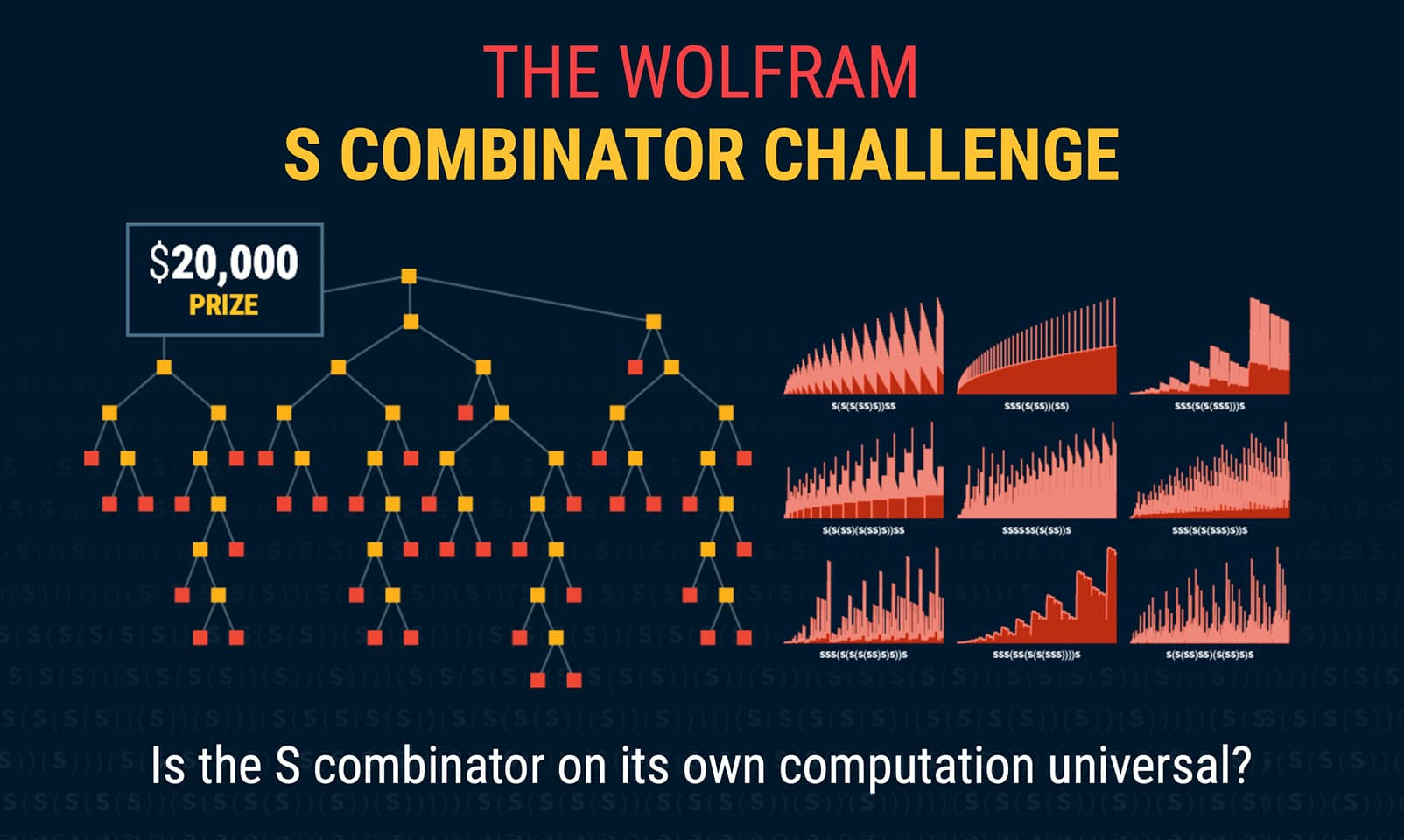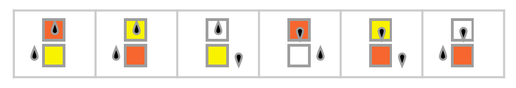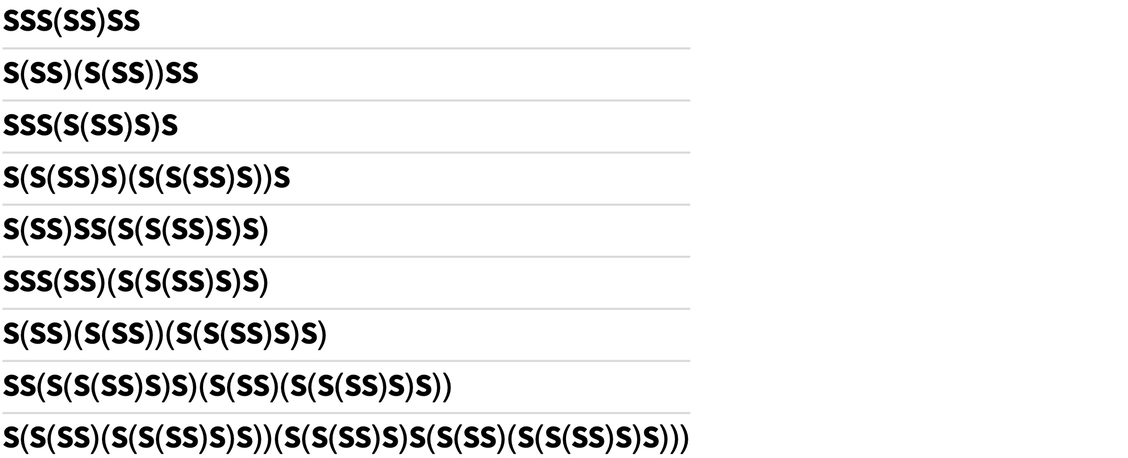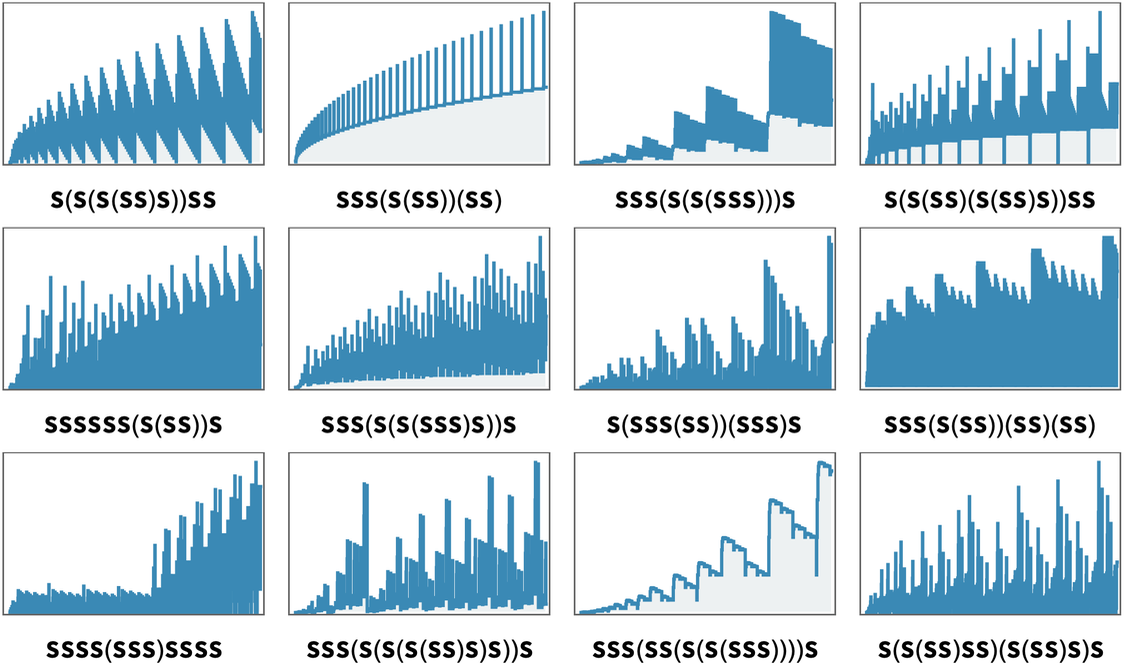1920, 2020 and a \$20,000 Prize: Announcing the S Combinator Challenge

# 1920, 2020 and a \$20,000 Prize: Announcing the S Combinator Challenge## Hiding in Plain Sight for a Century?

On December 7, 1920, Moses Schönfinkel introduced the S and K combinators—and in doing so provided the first explicit example of a system capable of what we now call universal computation. A hundred years later—as I prepared to celebrate the centenary of combinators—I decided it was time to try using modern computational methods to see what we could now learn about combinators. And in doing this, I got a surprise.

It’s already remarkable that S and K yield universal computation. But from my explorations I began to think that something even more remarkable might be true, and that in fact S alone might be sufficient to achieve universal computation. Or in other words, that just applying the rule

S f g xf[x][g[x]]

over and over again might be all that’s needed to do any computation that can be done.

I don’t know for sure that this is true, though I’ve amassed empirical evidence that seems to point in this direction. And today I’m announcing a prize of \$20,000 (yes, the “20” goes with the 1920 invention of combinators, and the 2020 making of my conjecture) for proving—or disproving—that the S combinator alone can support universal computation.

Why is it important to know? Obviously it’ll be neat if it turns out that hiding in plain sight for a century has been an even simpler basis for universal computation than we ever knew. But more than that, determining whether the S combinator alone is universal will provide an important additional data point in the effort to map out just where the threshold of universal computation lies.

Practical computers have complicated and carefully designed CPUs. But is that complexity really needed to support universal computation? My explorations in the computational universe over the past 40 years have led me to the conclusion that it absolutely is not, and that in fact even among systems with the very simplest rules, universal computation is actually quite ubiquitous.

I’ve developed a very general principle that I call the Principle of Computational Equivalence that implies that pretty much whenever we see behavior that isn’t in some sense obviously simple, then it will actually correspond to a computation that’s equivalent in sophistication to any other computation. And one of the many consequences of this principle is that it says computation universality should be ubiquitous.

For more than 30 years I’ve been pushing to get explicit evidence about this, by proving (or disproving) that particular systems are computation universal. And from this have come two very excellent examples that help validate the Principle of Computational Equivalence: the rule 110 cellular automaton✕ `RulePlot[CellularAutomaton]`

and the 2,3 Turing machine:✕ `RulePlot[TuringMachine[{596440, 2, 3}]]`

Both these systems I identified as the simplest of their type that could conceivably be computation universal—and both I expected would actually be computation universal.

But in both cases it was hard work to come up with an explicit proof. In the first case, the proof was part of my book A New Kind of Science (with much of the heavy lifting done by a then-research assistant of mine). In the second case, I’d already “made the conjecture” in A New Kind of Science, but in 2007 I decided to put up a prize for actually resolving it. And I was very pleased when, after just a few months, a proof was found, and the prize was won.

So now I’m hoping something similar will happen in the S combinator case. I’m expecting that it will turn out to be computation universal, but it would perhaps be even more interesting if it was proved not to be.

In the past one might have viewed proving that a simple system is (or is not) computation universal as a curiosity—something like solving a specific mathematical puzzle. But the Principle of Computational Equivalence—and all the science I’ve done as a result of exploring the computational universe—now places such a question in a broader context, and shows that far from being a curiosity, it’s actually quite central.

For example, with computation universality comes computational irreducibility, and undecidability. And to know how common these are in systems in nature, in mathematics and in technology has great fundamental and practical significance for when predictions can be made, and what kinds of questions can be answered.

Identifying the threshold of computation universality also has direct implications for making a molecular-scale computer, as well, for example, as in computer security, for knowing whether some part of some program is actually universal, so it can be programmed to do something bad.

## The Basic Setup

In the usual S, K combinator setup one does computations by setting up an initial combinator expression that encodes the computation one wants to do, then one repeatedly applies the combinator rules until one gets to a fixed point that can be interpreted as the result of the computation. Of course, not all computations halt, and for computations that don’t halt, no fixed point will ever be reached.

With the S combinator alone a direct input → output representation of computations won’t work. The reason is that there is an elaborate, but finite, characterization of every S combinator expression that halts, and the existence of this characterization implies that halting S combinator evolutions don’t have enough richness to represent arbitrary computations.

There are, however, plenty of non-halting S combinator evolutions. Here’s the very simplest of them:✕ ```ResourceFunction["CombinatorEvolutionPlot"][ Text[ResourceFunction["CombinatorPlot"][ ReplaceAll[#, {s -> CombinatorS, k -> CombinatorK}], "CharactersLeftAssociative", "SKGlyphs" -> {s, k}, "ApplicationGlyph" -> "\[NegativeVeryThinSpace]"]] & /@ ResourceFunction["CombinatorEvolveList"][s[s][s][s[s]][s][s], 8, "SKGlyphs" -> {s, k}], "StatesDisplay"]```

And here are examples of the sequences of sizes of expressions obtained in various such evolutions:✕ ```Grid[Partition[ Labeled[ListStepPlot[ Differences[ ResourceFunction["SKCombinatorLeftmostOutermostLeafCounts"][#, 1000, "SKGlyphs" -> {s, k}]], ImageSize -> 130, PlotRange -> All, Frame -> True, FrameTicks -> None, Filling -> Axis, FillingStyle -> Hue[0.56, 0.02, 0.9500000000000001], PlotStyle -> Hue[0.56, 0.68, 0.71]], Text[Style[ResourceFunction["CombinatorTraditionalForm"][#], 12]]] & /@ {s[s[s[s[s]][s]]][s][s], s[s][s][s[s[s]]][s[s]], s[s][s][s[s[s[s][s]]]][s], s[s[s[s]][s[s[s]][s]]][s][s], s[s][s][s][s][s][s[s[s]]][s], s[s][s][s[s[s[s][s]][s]]][s], s[s[s][s][s[s]]][s[s][s]][s], s[s][s][s[s[s]]][s[s]][s[s]], s[s][s][s][s[s][s]][s][s][s][s], s[s][s][s[s[s[s[s]][s]][s]]][s], s[s][s][s[s][s[s[s[s][s]]]]][s], s[s[s[s]][s][s]][s[s[s]][s]][s]}, 4]]```

And potentially it’s possible to do computations by “piggy-backing” on these non-halting evolutions. Start with a specification of the computation you want to do. Now encode it as an initial condition for one of the infinite number of possible non-halting combinator evolutions. Then run the combinator evolution until some particular feature occurs. Then decode from the results at that stage the output from the computation.

To prove that the S combinator is capable of supporting universal computation, one must show that something like this will work for any possible computation one wants to do. Or, in other words, one must show that an S combinator system can emulate some other system (say the S, K combinator one) that is already known to be universal. And by “emulate”, what we mean here is that a suitable “encoder”, “detector” and “decoder” can be found that will allow any evolution in the system being emulated to be mapped to a corresponding evolution in the S combinator system.

There is an important caveat, however: one has to be sure that the encoder, detector and decoder are not themselves capable of universal computation. If one’s lucky, these will operate in sufficiently straightforward ways so that it’ll be obvious they’re not universal. But in general it’ll be nontrivial to demonstrate that they’re not universal. Depending on the setup, one approach may be to show that for all inputs they must halt in some bounded time.

OK, so what if the S combinator system is not computation universal? How could one show that? One possibility would be to demonstrate that all, even infinite, evolutions have only a finite, or essentially finite, number of distinct forms. Another would be to establish that any “modulation” of such evolution must always die out in finite time, so that it must in effect correspond to a computation that halts.

A slightly different approach would be to show that S combinator evolution can be “solved” to the point where its properties after any number of steps can be determined by some bounded computation.

We’ve been talking about “doing computations” with combinators. But there’s a subtlety with that. Because there are in general many different possible evaluation orders for the combinator evolution. If the evolution halts, then it’s guaranteed that it’ll always reach the same fixed point, regardless of the order in which the evaluation was done. But if it doesn’t halt, there’s in general a whole multiway graph of possible sequences of results.

So what does computation universality then mean? As I’ve discussed elsewhere, a strong potential definition is to ask that the multiway system generated by the combinator system can effectively emulate the multiway system generated, say, by a multiway (or nondeterministic) Turing machine. But a weaker alternative definition might just be to ask that one can find some path (i.e. some evaluation order) that can perform any given (deterministic) computation. Or one might for example have detector and decoder functions that can work on some—or all—branches, somehow always being able to reproduce the computation one wants.

## The Operation of the S Combinator Challenge

I don’t know how long it’s going to take, or how hard it’s going to be, but I’m hoping that someday a message will arrive that successfully resolves the S Combinator Challenge. What will the message have to contain?

The best case as far as I am concerned is specific Wolfram Language code that implements the solution. If the S combinator is in fact universal, then the code should provide a “compiler” that takes, say, a Turing machine or cellular automaton specification, and generates an S combinator initial condition, together with code for the “detector” and “decoder”. But how will we validate that this code is “correct”?

It might conceivably be simple enough that it’s amenable to direct human analysis. But more likely it’s going to require some level of automated analysis. At first one might just do explicit testing. But then one might for example try to generate an automated proof that the detector and decoder always halt, and even perhaps determine an upper bound on how long this can take.

If the S combinator is not universal, one might show this by having code that can “jump ahead” by any number of steps and explicitly determine the outcome, but that itself always halts. Or maybe one might have code that effectively implements what happens to any “modulation” of S combinator evolution—and then prove that this code leads to behavior that, say, always halts, or otherwise ultimately shows complete regularity.

But maybe one won’t have explicit code that implements a solution, and instead one will only have an abstract proof of what’s possible. This could conceivably be in the form of a standard human-oriented mathematical proof. But I think it’s more likely it will be complicated enough that it has to be presented in a systematic computational way. And in the end, if it’s a proof it’ll have to start from certain axioms.

What axioms can be used? One would hope that the “standard axioms of mathematics” will be enough. Perhaps only the Peano axioms will be needed. But I wouldn’t be surprised if transfinite induction were needed, requiring the axioms of set theory. And of course there are weird cases that could arise, where the proof will be possible, say, with one assumption about the Continuum Hypothesis, but not with the other.

There are other weird things that could happen. For example, it might be possible to show that the complete infinite structure obtained by running the combinator evolution for an infinite time could reproduce any computation, but one might not know whether this is possible with finite evolution. Or it might be that one could only make a construction if one had an infinite tree (say specified by a transfinite number) as the initial condition.

It’s also conceivable that the behavior of the S combinator could correspond to an “intermediate degree” of computational capability—and have undecidable features, but not be universal. I don’t think this will happen, but if it does, I would consider it a resolution of the S Combinator Challenge.

And then, of course, there’s the unexpected. And in my experience of exploring the computational universe over the past 40 years, that’s something one encounters with remarkable frequency. A corner case one didn’t anticipate. An intermediate situation that didn’t seem possible. A new form of behavior one never imagined. Sometimes these are the most interesting things to find. But inevitably they make giving a cut-and-dried definition of what’s needed for the S Combinator Challenge difficult.

It’s worth remembering, though, that the S Combinator Challenge is about answering the human-stated question “Is the S combinator computation universal?”. And in the end it may take humans to determine whether some particular potential solution really does answer that question or not.

In a sense the S Combinator Challenge is already a 100-year story. But I’m certainly hoping that it’ll be resolved in far less than 100 more years. And that in doing so we’ll learn important things about the remarkable world of combinators, and the computational universe in general.